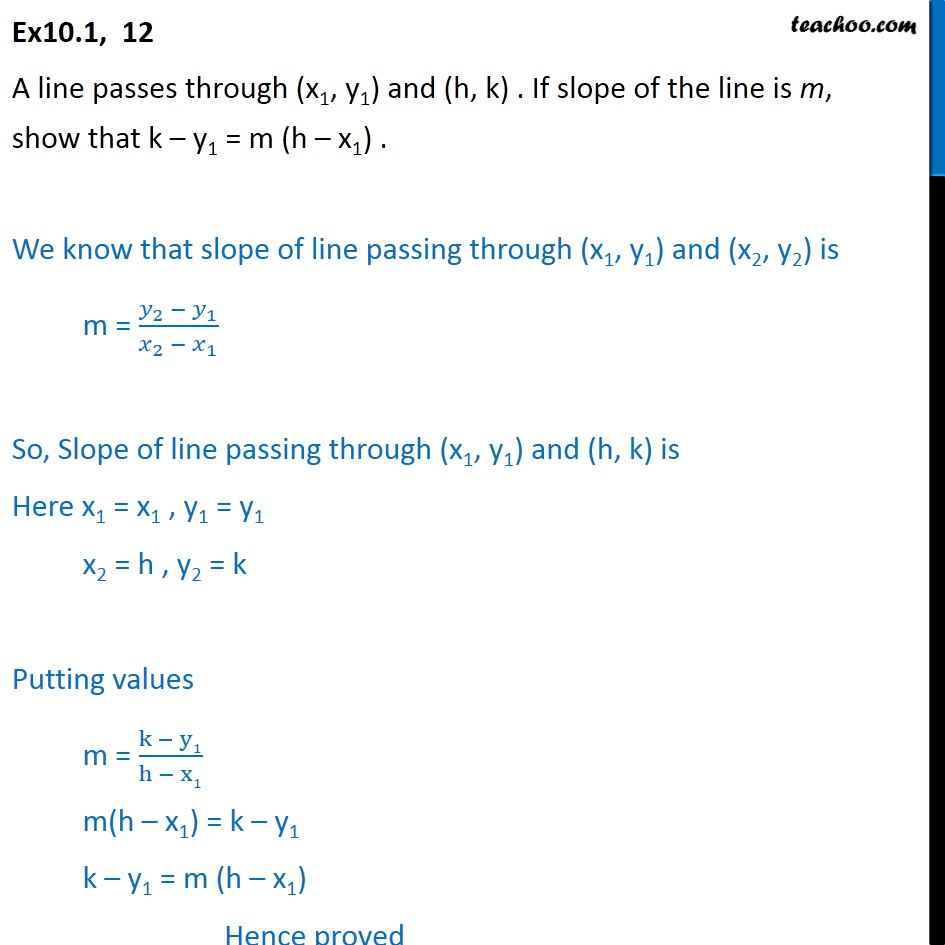Ex 9.1

Chapter 9 Class 11 Straight Lines
Serial order wiseLearn in your speed, with individual attention - Teachoo Maths 1-on-1 Class

### Transcript

Ex10.1, 12 A line passes through (x1, y1) and (h, k) . If slope of the line is m, show that k y1 = m (h x1) . We know that slope of line passing through (x1, y1) and (x2, y2) is m = ( _2 _1)/( _2 _1 ) So, Slope of line passing through (x1, y1) and (h, k) is Here x1 = x1 , y1 = y1 x2 = h , y2 = k Putting values m = (k y1)/(h x1) m(h x1) = k y1 k y1 = m (h x1) Hence proved